# Effective Interest Rate Calculator

Interest rates are more than meets the eye. Skip the guesswork and see how much you'll actually earn with this effective interest rate calculator.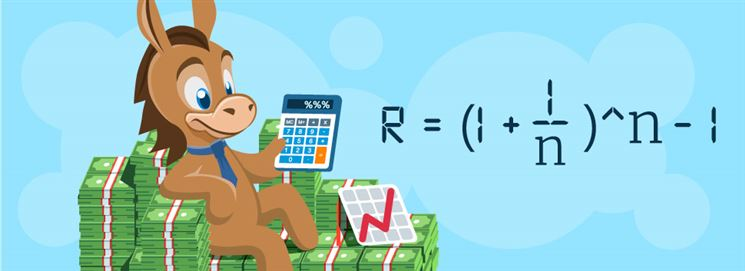Effective Interest Rate

As a smart investor, you know that it pays to compare effective interest rate.

This tells you how much an investment actually earns over time, with compounding factored in.

But don't worry about doing the math yourself. With this calculator, you'll know:

• The effective interest rates of two different investments
• How much they'll earn per compounding period
• How much they'll earn over 3 years

Find out how to calculate effective interest rate in this guide, plus more tips for investing wisely.

### What is effective interest rate?

Effective interest rate is the actual rate of return you get on an interest-earning investment. Effective interest factors both compound frequency and time into the calculation.

Effective interest rate is different from the nominal interest rate, which doesn't include any compounding or the passage of time.

How long is your investment time horizon?

Nominal interest rate is usually what you see advertised for investments, like CDs or high-yield savings accounts. But you're better off knowing the effective interest rate to evaluate how good the investment is.

Learn how to calculate the effective interest rate below.

### How do you calculate effective interest rate?

To calculate the effective interest rates for two different investments, follow the steps below.

1. Enter the nominal (advertised) interest rate of the investment.

2. Add the compound frequency of that investment.

3. Then, enter the nominal (advertised) interest rate of a different investment.

4. Add the compound frequency of the second investment.

5. Finally, hit "Calculate".

The calculator will show you the effective interest rate for both investments, plus the effective rate they'll earn over 3 years. This can help you determine which is the more worthwhile investment.

### Effective interest rate formula

The formula for effective interest rate is:

r = (1 + i/n)^n - 1

• r is the effective interest rate
• i is the nominal interest rate advertised on an investment
• n is the number of compounding per year

Here is an example of an investment with the advertised interest rate of 10%. Let's calculate the Effective Interest Rate if the investment is to be compounded 2x yearly.

Semi-annually
Nominal Rate / Advertised Interest Rate (i) = 10%
Number of Compounding Per Year (n) = 2

Effective Interest Rate is calculated using the formula:
Effective Interest Rate = (1 + i/n)n - 1
Effective Interest Rate = (1 + 10%/2)2 - 1
Effective Interest Rate = 10.25%

Effective Interest Rate Table
The table shows the effective interest rate based on the frequency of compounding for the nominal rates from 1% - 10%.

Nominal RateSemi-AnnuallyQuarterlyMonthlyDaily
1%1.002%1.004%1.005%1.005%
2%2.010%2.015%2.018%2.020%
3%3.022%3.034%3.042%3.045%
4%4.040%4.060%4.074%4.081%
5%5.062%5.095%5.116%5.127%
6%6.090%6.136%6.168%6.183%
7%7.122%7.186%7.229%7.250%
8%8.160%8.243%8.300%8.328%
9%9.202%9.308%9.381%9.416%
10%10.250%10.381%10.471%10.516%

How many compounding periods per year:
Semi-annually = 2x yearly
Quarterly = 4x yearly
Monthly = 12x yearly
Semi-monthly = 24x yearly
Daily = 365x yearly

### When to use effective interest rate

Effective interest rate is a crucial factor in evaluating investments and loans.

This will give you a more accurate idea of how much you can earn or how much you'll be charged. While it's always a helpful figure to know, it's especially important for loans.

This is because the effective interest rate is normally higher than the nominal interest rate. By looking at the nominal rate only, you're underestimating how much a loan will cost you over time.

Thinking about taking out a loan? Once you have the effective interest rate, try using one of our calculators to estimate your monthly payments:

### Different types of interest

Whether you're saving, investing, or taking out a loan, it pays to know the difference between interest rates. Find out how each type of interest differs below:

Effective interest rate
Effective interest rate is the actual rate of return you get on an interest-earning investment, with time and compound frequency factored in.

Nominal interest rate
Also called the coupon rate, this is the stated interest rate on an investment or loan, without any compounding or fees factored in.

Real interest rate
The real interest rate is the interest rate on an investment or loan with inflation factored in. Since inflation is unknown for future points in time, real interest rates are considered an estimate.

Annual percentage yield
Like effective interest rates, APY is the annual interest generated on an investment or loan with compounding factored in.

Annual percentage rate
APR is the annual interest generated on an investment or loan without compounding factored in.

Compound interest
Compound interest isn't like the other interest rates listed above - it's not a rate advertised on an investment or loan.

Compound interest is when you earn interest on the interest you've already earned. How often your investment earns this interest is referred to as the compounding period.

For example, if your investment compounds monthly, the compound interest is calculated and added to your balance every month.

### How to make smart investments

Knowing the effective interest rate is just one part of evaluating an investment. Here are some other tips to help you invest wisely.

• Find your perfect investing strategy. Are you more hands-on? Or do you want to let an expert take control?

Think about what makes the most sense for your schedule and preferences and seek platforms that work for what you need.

Passive investors might like a robo-advisor, while hands-on types can cherry-pick their investments with platforms like Robinhood, Public, or Webull.

• Budget your investments. Budgeting isn't just for bills and savings, it pays to budget your investments each month, too.

If you don't have a budget already, consider the 50-30-20 budget. This offers an easy way to divide up your needs, wants, and other important expenses.

Or try an online budgeting tool that matches your budgeting philosophy.

• Keep track of your investments. This is especially important if you have investing accounts with multiple platforms. If you see all your investments in one place, you'll have a better understanding of where your money is going.

Try an investment tracking software, or a stock tracking app to keep tabs on your returns over time.

### Bottom Line

Now that you know the full story of effective interest rates, you're in a better position to evaluate potential investments and pick the best ones for your portfolio.

Don't forget to use it for loans, as well. By looking at the effective interest rate instead of the nominal interest rate, you'll understand how much the loan will truly cost you.

Holly Zorbas is a assistant editor at CreditDonkey, a personal finance comparison and reviews website. Write to Holly Zorbas at holly.zorbas@creditdonkey.com. Follow us on Twitter and Facebook for our latest posts.

Note: This website is made possible through financial relationships with some of the products and services mentioned on this site. We may receive compensation if you shop through links in our content. You do not have to use our links, but you help support CreditDonkey if you do.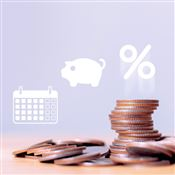#### How Much Interest Does \$1000 Earn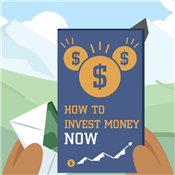### How to Invest Money#### Average Net Worth by Age#### Savings Goal Calculator### Budget Calculator### Get a Free Stock (worth between \$2.50 and \$225)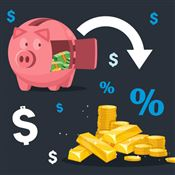#### Rollover 401k to Gold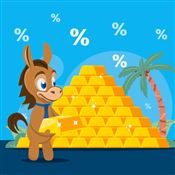#### Orion Metal Exchange Review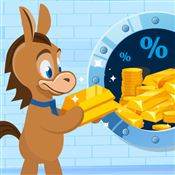#### Oxford Gold Group Review#### Home Storage Gold IRA#### Birch Gold Group Review

How long is your investment time horizon?
36% Less than 1 year
36% 1 to 5 years
9% 6 to 10 years
18% More than 10 years
Source: CreditDonkey. Totals may not add to 100% due to rounding.

### Free Stocks

Looking for ways to get stocks without spending a fortune? You may be surprised to find there are places where you can get stocks for free.